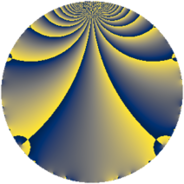# Properties

 Label 555.2.gLevel $555$ Weight $2$ Character orbit 555.g Rep. character $\chi_{555}(184,\cdot)$ Character field $\Q$ Dimension $40$ Newform subspaces $1$ Sturm bound $152$ Trace bound $0$

# Learn more about

## Defining parameters

 Level: $$N$$ $$=$$ $$555 = 3 \cdot 5 \cdot 37$$ Weight: $$k$$ $$=$$ $$2$$ Character orbit: $$[\chi]$$ $$=$$ 555.g (of order $$2$$ and degree $$1$$) Character conductor: $$\operatorname{cond}(\chi)$$ $$=$$ $$185$$ Character field: $$\Q$$ Newform subspaces: $$1$$ Sturm bound: $$152$$ Trace bound: $$0$$

## Dimensions

The following table gives the dimensions of various subspaces of $$M_{2}(555, [\chi])$$.

Total New Old
Modular forms 80 40 40
Cusp forms 72 40 32
Eisenstein series 8 0 8

## Trace form

 $$40q + 44q^{4} - 40q^{9} + O(q^{10})$$ $$40q + 44q^{4} - 40q^{9} + 4q^{10} + 8q^{11} + 52q^{16} - 16q^{21} + 8q^{25} - 16q^{26} + 16q^{30} - 32q^{34} - 44q^{36} - 28q^{40} + 8q^{41} + 16q^{44} - 8q^{46} - 24q^{49} + 92q^{64} - 48q^{65} - 56q^{70} + 24q^{71} - 68q^{74} + 8q^{75} + 40q^{81} - 16q^{84} - 64q^{85} + 80q^{86} - 4q^{90} + 32q^{95} - 8q^{99} + O(q^{100})$$

## Decomposition of $$S_{2}^{\mathrm{new}}(555, [\chi])$$ into newform subspaces

Label Dim. $$A$$ Field CM Traces $q$-expansion
$$a_2$$ $$a_3$$ $$a_5$$ $$a_7$$
555.2.g.a $$40$$ $$4.432$$ None $$0$$ $$0$$ $$0$$ $$0$$

## Decomposition of $$S_{2}^{\mathrm{old}}(555, [\chi])$$ into lower level spaces

$$S_{2}^{\mathrm{old}}(555, [\chi]) \cong$$ $$S_{2}^{\mathrm{new}}(185, [\chi])$$$$^{\oplus 2}$$